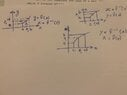# Inverse Functions

Consider the case of a real function f for which f inverse exists.

1) We we are not used to having the y-axis (vertical axis) to denote the independent variable which it does in x=f-1(y). We rotate the system through positive 90 degree and reflect about the vertical to change the sense of the axis. One further adjustment to make is to relabel the horizontal axis x and the vertical axis y (the names we give to the axes are immaterial).

If we accept the convention that the independent variable must be always plotted along the horizontal axis in the left right sense and the independent variable be called x. Then the first figure on the top left is the appropriate graph of y=f(x) and the bottom figure would be the appropriate graph for y=f^-1(x). Which figures are appropriate for these relations x=f(y) and x=f^-1(y)?

I think x=f(y) denotes the relation for the top left figure and x=f^-1(y) for the bottom figure because names to the input and output are irrelevant.

#### Attachments

•f8f2674e-5cf1-4c78-b783-6386f9f79207.jpg
25.1 KB · Views: 45
Last edited:

Homework Helper
Gold Member
2022 Award
Is there a question here?

Its stated in the study notes I am studying from that f(x)=y and f(y)=x are obtained from one another by merely reversing the roles of input and output. How is this possible? Arent both functions equal the difference between them two is what we choose to name as the input

Homework Helper
Gold Member
2022 Award
Its stated in the study notes I am studying from that f(x)=y and f(y)=x are obtained from one another by merely reversing the roles of input and output. How is this possible? Arent both functions equal the difference between them two is what we choose to name as the input
If you draw a graph of ##y = f(x)## and then rotate the graph by 90 degrees counter-clockwise, then that is the graph of ##x = f^{-1}(y)##. It's not quite right, because the y-axis goes off to the left, rather then the right, so you also have to reflect the graph in what is now the vertical x-axis. That's just left/right convention.

Take as an example the graph of ##y = x^3##. The point ##(x=2, y=8)## is on the graph. If we rotate the graph then that becomes the point ##(y = 8, x=2)## and we see that ##x = y^{1/3}##. And, of course, the cube and cube root are inverse functions.

••